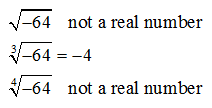## Algebra

Showing posts with label radicals. Show all posts
Showing posts with label radicals. Show all posts

### Radicals Including Square and Cube Roots

We have seen square roots before, however, we will go into a bit more depth here.

Also, we will generalize this concept and introduce nth roots. The definition of the principal (non-negative) square root follows.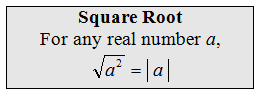Simplify.Not all square roots work out so nicely.  If trying to simplify a square root with a radicand that is not a perfect square, we can find the exact answer using the following properties.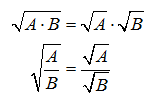Here A and B are non-negative real numbers and B is not equal to zero. Approximations are made using a calculator.

Give the exact answer and approximate to the nearest hundredth.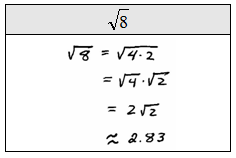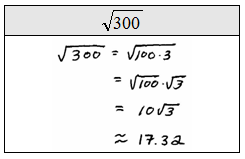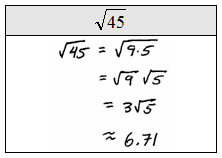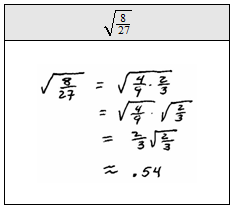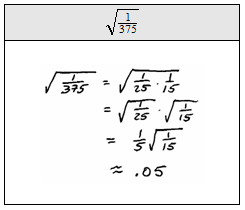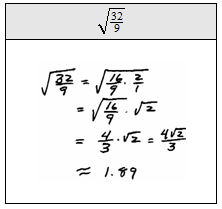This idea can be extended to any positive integer index n.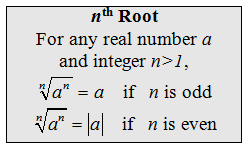For 3rd roots, or cube roots, the question is “what raised to the 3rd power will produce the given number?" For example,becauseSimplify.Remember that if the radicand of an odd root is negative then the result will be a negative real number.  If the radicand of an even root is negative then the number is not real.